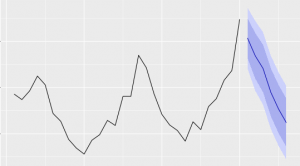Want to share your content on R-bloggers? click here if you have a blog, or here if you don't.The standard ARIMA (autoregressive integrated moving average) model allows to make forecasts based only on the past values of the forecast variable. The model assumes that future values of a variable linearly depend on its past values, as well as on the values of past (stochastic) shocks. The ARIMAX model is an extended version of the ARIMA model. It includes also other independent (predictor) variables. The model is also referred to as the vector ARIMA or the dynamic regression model.
The ARIMAX model is similar to a multivariate regression model, but allows to take advantage of autocorrelation that may be present in residuals of the regression to improve the accuracy of a forecast.
This set of exercises provides a practice in using the auto.arima function from the forecast package to make forecasts with the ARIMAX model. A function from the lmtest package is also used to check the statisical significance of regression coeffcients.
The exercises make use of the Icecream dataset from the Ecdat package. The dataset contains the following variables:

• ice cream consumption in the USA (in pints, per capita),
• average family income per week (in USD),
• price of ice cream (per pint), and
• average temperature (in Fahrenheit).

The number of observations is 30. They correspond to four-weekly periods in the span from March 18, 1951 to July 11, 1953 (download here).

For other parts of the series follow the tag forecasting.

Answers to the exercises are available here.

Exercise 1
Load the dataset, and plot the variables cons (ice cream consumption), temp (temperature), and income.

Exercise 2
Estimate an ARIMA model for the data on ice cream consumption using the auto.arima function. Then pass the model as input to the forecast function to get a forecast for the next 6 periods (both functions are from the forecast package).

Exercise 3
Plot the obtained forecast with the autoplot.forecast function from the forecast package.

Exercise 4
Use the accuracy function from the forecast package to find the mean absolute scaled error (MASE) of the fitted ARIMA model.

Exercise 5
Estimate an extended ARIMA model for the consumption data with the temperature variable as an additional regressor (using the auto.arima function). Then make a forecast for the next 6 periods (note that this forecast requires an assumption about the expected temperature; assume that the temperature for the next 6 periods will be represented by the following vector:  fcast_temp <- c(70.5, 66, 60.5, 45.5, 36, 28)).
Plot the obtained forecast.

Learn more about Forecasting in the online course Time Series Analysis and Forecasting in R. In this course you will learn how to:

• A complete introduction on Forecasting
• Work thru an exponentional smoothing instruction
• And much more

Exercise 6
Print summary of the obtained forecast. Find the coefficient for the temperature variable, its standard error, and the MASE of the forecast. Compare the MASE with the one of the initial forecast.

Exercise 7
Check the statistical significance of the temperature variable coefficient using the the coeftest function from the lmtest package. Is the coefficient statistically significant at 5% level?

Exercise 8
The function that estimates the ARIMA model can input more additional regressors, but only in the form of a matrix. Create a matrix with the following columns:

1. values of the temperature variable,
2. values of the income variable,
3. values of the income variable lagged one period,
4. values of the income variable lagged two periods.

Print the matrix.
Note: the last three columns can be created by prepending two NA‘s to the vector of values of the income variable, and using the obtained vector as an input to the embed function (with the dimension parameter equal to the number of columns to be created).

Exercise 9
Use the obtained matrix to fit three extended ARIMA models that use the following variables as additional regressors:

1. temperature, income,
2. temperature, income at lags 0, 1,
3. temperature, income at lags 0, 1, 2.

Examine the summary for each model, and find the model with the lowest value of the Akaike information criterion (AIC).
Note that the AIC cannot be used for comparison of ARIMA models with different orders of integration (expressed by the middle terms in the model specifications) because of a difference in the number of observations. For example, an AIC value from a non-differenced model, ARIMA (p, 0, q), cannot be compared to the corresponding value of a differenced model, ARIMA (p, 1, q).

Exercise 10
Use the model found in the previous exercise to make a forecast for the next 6 periods, and plot the forecast. (The forecast requires a matrix of the expected temperature and income for the next 6 periods; create the matrix using the fcast_temp variable, and the following values for expected income: 91, 91, 93, 96, 96, 96).
Find the mean absolute scaled error of the model, and compare it with the ones from the first two models in this exercise set.